# Solutions to SQA examination

### Paper II Solutions

```1.a.	The ball is in free fall for the first 0.5s.

u = 0m/s
v = -4.5m/s
t = 0.5s
a = ?

a = (v-u)/t
a = (-4.5-0)/0.5
a = -9.0m/s/s

b.	DEk = Ekafter-Ekbefore
DEk = 1/2(mv2-mu2)
2DEk = m(v2-u2)
2DEk/(v2-u2) = m
m = 2DEk/(v2-u2)
m = 2x-1.7/(42-[-4.52])
m = -3.4/(16-20.25)
m = 0.8kg

c.	DP = mv-mu
DP = m(v-u)
DP = 0.8(4-[-4.5])
DP = 6.8kgm/s

d.	The time that the ball is in contact with the ground(tc) is 0.5s.
F = DP/tc
F = 6.8/0.5
F = 13.6N

2.a.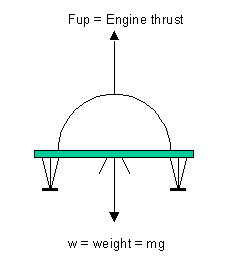w = ?
m = 15000
g = 1.6N/kg

w = mg
w = 15000x1.6
w = 24000N

The upward force(Fup) is equal in magnitude to the weight
and acts in the opposite direction. There is no unbalanced force
which means the craft must be moving at a constant speed and be moving
in a straight line.

b.i.	Funbalanced = Fup - w
Funbalanced = 25500 - 24000
Funbalanced = 1500N

a = Funbalanced/m
a = 1500/15000
a = 0.1m/s/s upwards

This means that the craft has an upward deceleration of -0.1m/s/s.

b.ii.	a = 0.1m/s/s
t = 18s
u = -2.0m/s
v = ?

v = u + at
v = -2.0 + 0.1x18
v = -0.2m/s

b.iii.v2 = u2 + 2as
s = (v2-u2)/2a
s = ([-0.2]2-[-2]2)/2x0.1
s = (0.04-4)/0.2
s = -3.96/0.2
s = -19.8m

This displacement is equal to that which the craft has descended from.
This means the craft must have been 19.8m above the surface of the moon

3.a. 	 The law of conservation of linear momentum states that :
Pbefore = Pafter

b.i.	Before collision
Pbefore = mAuA + mBuB
Pbefore = 1400xuA + 1000x8
Pbefore = 1400uA + 8000

After collision
Pafter = mAvA + mBvB
vA=vB=v
Pafter = (mA + mB)v
Pafter = (1400 + 1000)15
Pafter = 36000kgm/s

Equate: Pbefore and Pafter
1400uA + 8000 = 36000kgm/s
1400uA = (36000-8000)/1400
uA = 20m/s

b.ii.	Consider the instant after the collision to be the starting point
for this calculation.

u = 15m/s
v = 0m/s
s = 20m
a = ?

v2 = u2 + 2as
a = (v2-u2)/2s
a = (02-152)/2x20
a = -225/40
a = -5.625m/s/s

Ffriction = Fun
Fun = ma
Fun = 2400x-5.625
acts to the LHS.

OR

The kinetic energy lost by the car is equal to the work done by
the frictional force over the 20m stopping distance.

Eklost = Ework

Eklost = Ekbefore - Ekafter
Eklost = (mv2 - mu2)/2
Eklost = m(v2 - u2)/2
Eklost = 2400(152 - 02)/2
Eklost = 27000J

Ework = Fd
F = Ework/d
F = 27000/20
F = 13500N

b.iii.Most of the kinetic energy will be converted into heat energy.

4.a.	P1 = 400kPa
V1 = 1000cm3
P2 = 250kPa
V2 = ?

P1V1 = P2V2
V2 = P1V1/P2
V2 = 400x1000/250
V2 = 1600cm3

b.	The pressure increases.
The volume remains constant.
The temperature increases.

c.	The starting pressure is that indicated by point B on the graph
and the final pressure is that indicated by point C. Over this
period the volume is constant.

T1 = 300K
P1 = 200kPa
T2 = ?
P2 = 500kPa

P1/T1 = P2/T2
T2 = P2(T1/P1)
T2 = 500(300/200)
T2 = 750K

5.a.i.	Q = CV
C = Q/V
C = 24x10-6/1.5
C = 16x10-6F
C = 16mF

a.ii.	Mean = total/N
Mean(C) = (16+18+20+16+15)/5
Mean(C) = 17mF

Random error = (max-min)/N
Random error(C) = (20-15)/5
Random error(C) = +-1mF

C = (17+-1)mF

a.iii.Measure the voltage across the capacitor directly by connecting
the voltmeter across it. Additionally, to prevent leakage through
the voltmeter it would be better to use an oscilloscope for this
purpose.

b.	The effective resistance of the capacitor in the circuit decreases
as the frequency of the supply increase. As the resistance decreases
the current in the circuit increases and the lamp glows more brightly.

6.a.	The electromotive force is the energy supplied by the power supply
to each coulomb of charge.

b.i.	P = I2R
I2 = P/R
I = (P/R)1/2
I = (8.0/0.32)1/2
I = 251/2
I = 5A

b.ii.	V = IR
V = 5x0.32
V = 1.6V

b.iii.Vr = emf - Vheater
Vr = 2.0 - 1.6
Vr = 0.4V

Vr = Ir
r = Vr/I
r = 0.4/5
r = 0.08W

c.	Two heaters in parallel will reduce the resistance of the circuit.
The current in the heater elements will increase which has the
effect of increasing the rate at which the water is heated.

The resistance of the two heaters in parallel can be calculated as
shown below:

Rp = Rheater/2
Rp = 0.32/2
Rp = 0.16W

Rtotal = Rp + r
Rtotal = 0.16 + 0.08
Rtotal = 0.24W

I = emf/Rtotal
I = 2.0/0.24
I = 8.33A

Vr = Ir
Vr = 8.33x0.08
Vr = 0.67V

Vheater = emf - Vr
Vheater = 2.0 - 0.67
Vheater = 1.33V

This is the voltage across each heater element.

Iheater = Vheater/Rheater
Iheater = 1.33/0.32
Iheater = 4.16A

The power developed in each heater can be calculated using:
P = I2R

P = 4.162x0.32
P = 5.5W

The two heating elements develop a total power of 11W.
This means that the water is heated about 1.4 times faster.

7.a.i.	The amplifier is working in inverting mode.

a.ii.	Gain = -Rfeedback/R1
Gain = -1x106/2x103
Gain = 500

a.iii.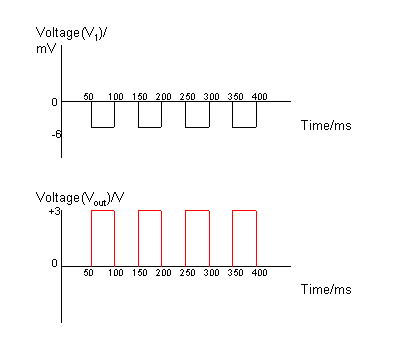a.iv.	The LED will pulse ON/OFF.
The LED will be on when Vout is positive.
The LED will be off when Vout is zero.

b.i.	The op-amp is working in differential mode.
The gain equation for the op-amp in this mode is:

Vout = (Rf/R1)(V2-V1)

b.ii.	Use the equation in b.ii. to calculate Vout. Take the
voltages V1 and V2 from the graphs given.
```
 Time interval/ms V2/mV V1/mV Vout/V 0-50 0 0 0 50-100 -6 -6 0 100-150 +6 0 +3 150-200 0 -6 +3 200-250 0 0 0 250-300 -6 -6 0 300-350 +6 0 +3 350-400 0 -6 +3
```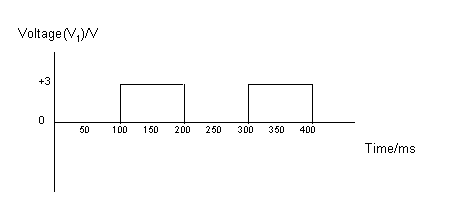8.a.	A ray of sunlight is made up of many different wavelengths that
is perceived as white light. However, each individual wavelength
represents a particular colour of light.
The diffraction grating diffracts the different wavelengths in
sunlight by different amounts: the longer wavelengths being
diffracted the most and the shorter wavelengths the least.
At position X on the screen the short wavelength violet light
has interfered constructively to produce a band of violet light.
At position Y on the screen the long wavelength red light
has interfered constructively to produce a band of red light.
Between X and Y the other colours of the visible spectrum produce
bands of indigo, blue, green, yellow and orange light.

b.i.	dsinq = nl

d = 1/N = 1/6x105 = 1.667x10-6m
q = ?
n = 1
l = 410nm = 410x10-9m

sinq = nl/d
sinq = 1x410x10-9/1.667x10-6
sinq = 0.246
q = sin-10.246
q = 14.2o

b.ii.	d = 1.667x10-6m
q = 14.2o + 9o = 23.2o
n = 1
l = ?

l = dsinq/n
l = 1.667x10-6sin(23.2o)/1
l = 656.7x10-9m = 656.7nm

c.	The diffraction grating will produce many spectra symmetrical about the
q = 0o line.
The prism produces one spectrum by refraction.
The relative positions of each colour of light produced
by refraction will be the reverse of that produced by the
diffraction grating, as blue light is refracted more than
red light.

9.a.	Monochromatic means the laser light has a single wavelength/frequency.
Coherent means the waves are in phase/step.

b.i.	DE = hf 	where DE = E2-E2
f = E2-E2/h
f = -4.67x10-20-(-6.55x10-20)/6.63x10-34
f = 452.5nm

b.ii.	The wavelength of the emitted radiation corresponds to the blue end of
the spectrum.

c.	The beam is intense because the energy is spread over a small area and
a large number of photons per second are emitted from the laser.

10.a.	Light incident on the pn-junction photodiode will increase the number
of electron hole pairs and consequently increase the conductivity of
the diode.

b.	The diode is operating in photoconductive mode.

c.	I1d12 = I2d22
I2 = I1d12/d22
I2 = 3.0x12/0.752
I2 = 5.33mA

11.a.i.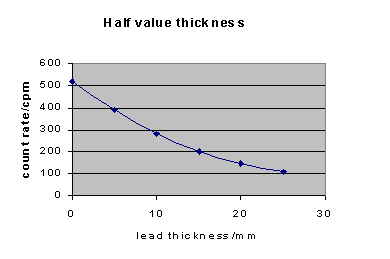a.ii.	Take the background count to be 20cpm.
Add this to the corrected count rate and plot as shown below.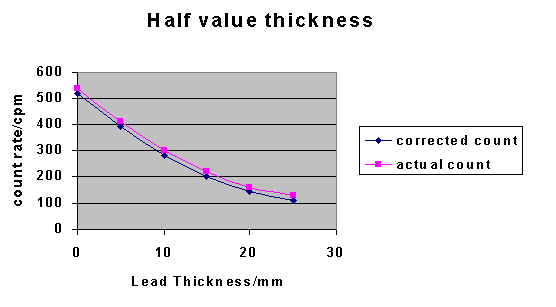b.	Time/years	Corrected Count Rate/cpm
0		520
5.25		260
11.5		130
16.75		65
21		32.5

After 21 years the count rate is 32.5cpm.

END OF QUESTION PAPER